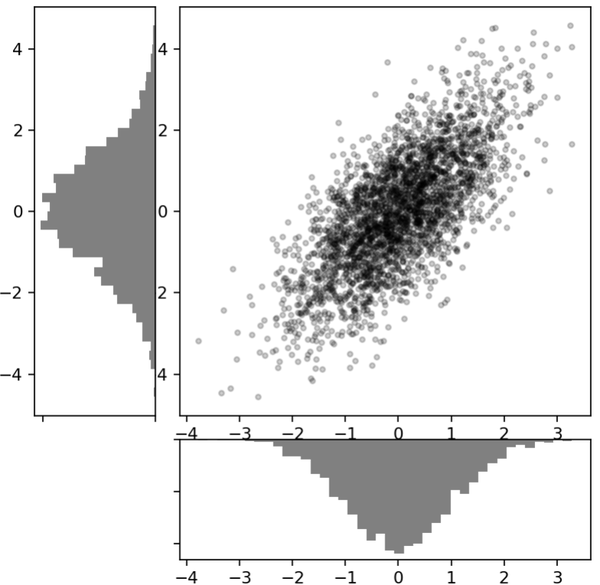# 多子图

### 一、手动创建子图

```ax1 = plt.axes()  # 使用默认配置，也就是布满整个画布
ax2 = plt.axes([0.65,0.65,0.2,0.2]) # 在右上角指定位置
```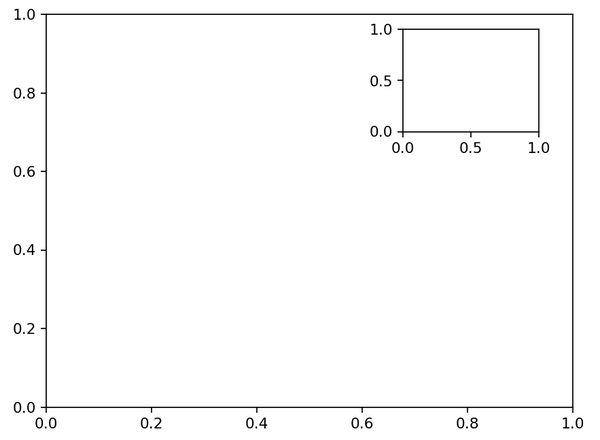```fig = plt.figure()

x = np.linspace(0,10)
ax1.plot(np.sin(x))
ax2.plot(np.cos(x))
```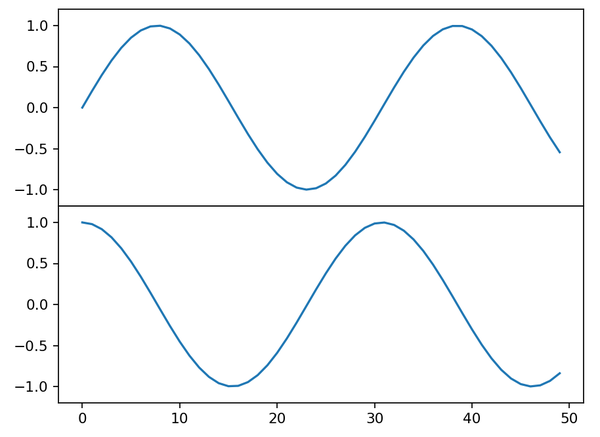### 二、 plt.subplot方法

subplot的方法接收三个整数参数，分别表示几行、几列、子图索引值。索引值从1开始，从左上角到右下角依次自增。

```for i in range(1, 7):  # 想想为什么是1-7
plt.subplot(2,3,i)
plt.text(0.5,0.5,str((2,3,i)), fontsize=16, ha='center')
```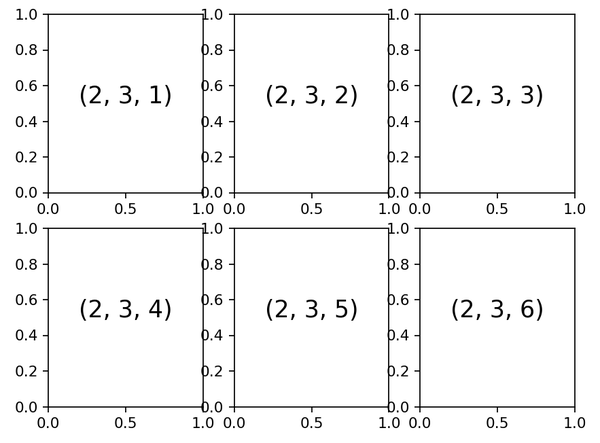```fig = plt.figure()
for i in range(1,7):
ax.text(0.5,0.5,str((2,3,i)), fontsize=16, ha='center')
```

### 三、 快速创建多子图

```fig, ax = plt.subplots(2,3,sharex='col', sharey='row')
```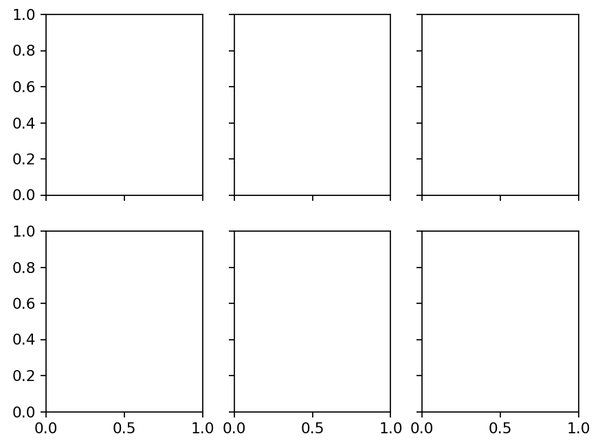```for i in range(2):
for j in range(3):
ax[i,j].text(0.5,0.5,str((2,3,i)), fontsize=16, ha='center')
```

### 四、GridSpec 复杂网格

```grid = plt.GridSpec(2,3,wspace=0.4,hspace=0.4) # 生成两行三列的网格

plt.subplot(grid[0,0]) # 将0，0的位置使用
plt.subplot(grid[0,1:]) # 同时占用第一行的第2列以后的位置
plt.subplot(grid[1,:2])
plt.subplot(grid[1,2])
```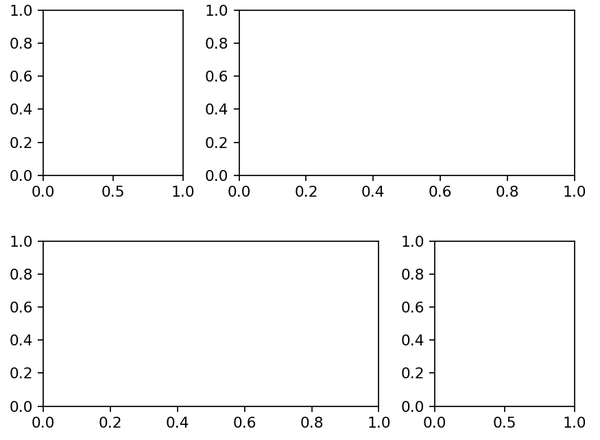```# 创建一些正态分布数据，这不是我们关心的内容
mean = [0, 0]
cov = [[1, 1], [1, 2]]
x, y = np.random.multivariate_normal(mean, cov, 3000).T

# 建立网格和子图
fig = plt.figure(figsize=(6, 6))
grid = plt.GridSpec(4, 4, hspace=0.2, wspace=0.2)
main_ax = fig.add_subplot(grid[:-1, 1:])  # 注意切片的方式
y_hist = fig.add_subplot(grid[:-1, 0], xticklabels=[], sharey=main_ax)
x_hist = fig.add_subplot(grid[-1, 1:], yticklabels=[], sharex=main_ax)

# 在主子图上绘制散点图
main_ax.plot(x, y, 'ok', markersize=3, alpha=0.2)

# 在附属子图上绘制直方图
x_hist.hist(x, 40, histtype='stepfilled',
orientation='vertical', color='gray')
x_hist.invert_yaxis() # 让y坐标轴的值由大到小，逆序

y_hist.hist(y, 40, histtype='stepfilled',
orientation='horizontal', color='gray')
y_hist.invert_xaxis() # 可以尝试不要这行，看看结果
```